# Mixed numbers + multiplication - math problems

#### Number of problems found: 61

• What is 11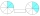What is 2/4 of 2 3/8?
• Countertop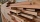Sam it is constructing he measures a rectangle area are on the countertop of 18 1/2 x 35 1/3” he will need to cut his area out in order to place his sink and the opening. What is the product of the two measurements round to the nearest whole?
• Nathan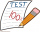Nathan is playing a trivia game. Each correct answer is worth 2 ½ points, and each incorrect answer is worth -¼ points. If he gets 35 questions correct and 32 questions incorrect, how many points does he have? A. 87.5 B. 79.5 C. 75 D. 8
• A land 2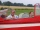A land owner owns two parcels of land measuring 4 2/5 hectares and 6 1/5 hectares. He sells 3/4 of the total land. How many hectares of the land are left?
• The product 3The product of the two numbers is 15 ⁵/₆. If one of the numbers is 5 ²/₃, find the other.
• AlexandraAlexandra made a rectangular quilt the measured 3 1/4. 2 3/4 feet in width. What is the area of the quilt in square feet? Write an equation to solve.
• The product 2The product of two functions is 10. If one of them is 2 1/3, find the other one.
• Simplify expression with mixedWhen (7¹/3 + 2¾)÷(2 + 2¼ × 1¹/3) is simplified the result is?
• Evaluate 17Evaluate 2x+6y when x=- 4/5 and y=1/3. Write your answer as a fraction or mixed number in simplest form.
• A burger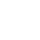The water starts with 41 1/4 cups of water how much water is left after ten 3 5/8 Scoops of water are removed?
• Evaluate fractionsThe difference  of 5 1/2 and 2/3 is added to the product of 5/6 and 1/2
• Evaluate mixed expressionsWhich of the following is equal to 4 and 2 over 3 divided by 3 and 1 over 2? A. 4 and 2 over 3 times 3 and 2 over 1 B. 14 over 3 times 2 over 7 C. 14 over 3 times 7 over 2 D. 42 over 3 times 2 over 31
• Long and wideWhat is the area of a rectangle that is 1 1/4 meters long and 3/4​ of a meter wide
• The length 6The length of 12 pipes is 10 1/2 meters. (1) find the length of one pipe (2) also find the length of 7 pipes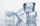Miriam is making 3 1/2 dozen cookies. If 2/7 of the cookies have icing, how many dozen cookies have icing?
• The length 5The length and breadth of rectangular field is 3 1/5 m  and 1 3/7 m. Find the area of rectangular field.
• Muffins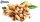One batch of walnut muffins uses 1 1/3 cups of walnuts. How many cups of walnut are needed to make 3 3/4  batches of muffins?
• Oil change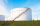A container holds 1/4 gallon of oil. Jack is changing the oil in his vehicles and needs 11 containers. How many gallons of oil does Jack need?
• Juila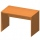Julia has a rectangular table that is 3 1/2 feet long and 1 3/4 feet wide. What is the area of Juila's table?
• JefferyJeffery is painting a wall the width of the wall is 9 feet the length of the was is 8 2/3 feet what is the area of the wall Jeffery wants to paint?

Do you have an exciting math question or word problem that you can't solve? Ask a question or post a math problem, and we can try to solve it.

We will send a solution to your e-mail address. Solved examples are also published here. Please enter the e-mail correctly and check whether you don't have a full mailbox.

Mixed numbers - math problems. Multiplication Problems.